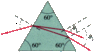# Indices of refraction when wavelengths are given

• ikihi

## Homework Statement

A parallel beam of light containing two wavelengths, λ1= 400 nm and λ2= 650 nm, enters the silicate flint glass (at a angle of 41 degrees, relative to normal) of an equilateral triangle (60 degrees at each angle) prism. At what angle, relative to the normal, does each beam leave the prism.

## Homework Equations

nair ⋅ sinθ1 = nprism ⋅ sinθ2

## The Attempt at a Solution

I don't get how to get the two different indices of refraction for the prism, one for each given wavelength.

#### Attachments

•24_49alt.gif
4.4 KB · Views: 379
Last edited:

••